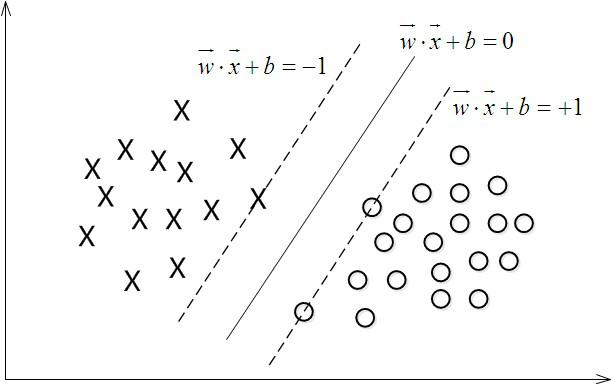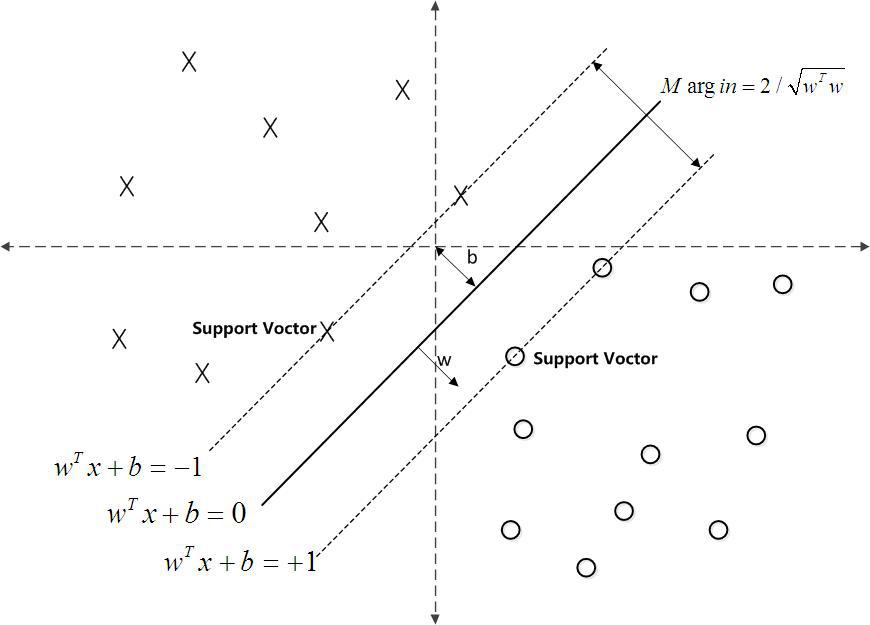## SVM 思路

### 1. 逻辑回归### 2. 函数间隔、集合间隔### 3. 最大化间隔分类器### 4. 拉格朗日乘子法

$$\mathcal{L}(\mathbf{w}, b, \alpha) = \frac{1}{2} ||\mathbf{w}||^2 - \sum_{i=1}^m \alpha_i (y_i \cdot (\mathbf{w} \mathbf{x}_i + b) - 1)$$

### 5. 对偶问题、KKT 条件

1. 对偶问题往往 更容易求解
2. 可以自然地 引入核函数，进而推广到非线性分类问题。

### 6. 求解对偶问题

1. 求 $\min$
2. 求 $\max$
3. 用 SMO 算法求解

### 8. 核函数

1. 如果数据在线性空间不可分，我们尝试将其映射到高维空间中。
2. 如果真的映射之后再计算，首先维度巨高，空间代价高，其次计算内积的计算量很大，时间代价很高。
3. 因此引入 核函数（Kernel Function）来 在低维空间中计算两个向量在高维空间中的映射的内积

$$\phi((x, y)) = (x^2, \sqrt 2 xy, y^2)$$

$$k(\vec{A}, \vec{B}) = (\vec{A}^\mathrm{T} \cdot \vec{B}^\mathrm{T})^2$$

### 9. 松弛变量

• 数据可能有异常点，无法完全线性可分，否则太过复杂甚至严重过拟合
• 因此 SVM 允许数据点在一定程度上偏离一下超平面，这里「允许的偏移程度」就是 松弛变量（Slack Variable），具体表现在原优化目标的不等式约束上。

## 旧文参考

1. linear classifier

$$f(x)=\langle W,x\rangle+b$$

1. lagrangian multiplier

\begin{align} W&=\sum_{i=1}^ma_iy_ix_i \\ f(x)&=\sum_{i=1}^ma_iy_i\langle x_i,x\rangle+b \end{align}

1. nolinear / high-dimensional space

\begin{align} x'&=\operatorname{highDim}(x) \\ x_i'&=\operatorname{highDim}(x_i) \\ f(x)&=\sum_{i=1}^ma_iy_i\langle x_i',x'\rangle+b \end{align}

1. kernel

\begin{align} K(a,b)&=\langle \operatorname{highDim}(a),\operatorname{highDim}(b)\rangle\\ f(x)&=\sum_{i=1}^ma_iy_i\langle x_i',x'\rangle+b \\ &=\sum_{i=1}^ma_iy_iK(x_i,x)+b \end{align}

## 常见问题

• SVM 可以做回归吗？
• 为什么说 SVM 适合用在小样本数据上？
• 为什么里面设定了 SVM 函数间隔为 1？
• SVM 会过拟合吗？受异常点的影响怎样？
• SVM 可以用在数据维度很大的推荐系统吗？
• 为什么可以用拉格朗日乘子？
• 为什么可以转化为对偶问题？